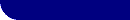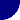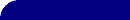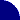# Other Circuit Elements - GerischerRESOURCES > EIS > DIFFUSION > GERISCHER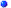The Gerischer circuit element appears in most of the equivalent circuit fitting programs, but many people are unsure of its meaning.##Other Circuit Elements - Gerischer

The Gerischer ( G ) circuit element was first derived for a preceding chemical reaction happening in the bulk solution. This is  the so called CE mechanism.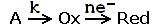A Gerischer  has also been used to model a porous electrode (ref 2).

On a Nyquist plot, it looks quite a lot like the O diffusion element (diffusion through a thin layer).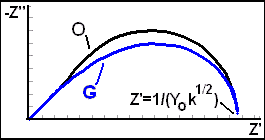A Nyquist plot for the G  element. It is not as high as an O element with the same intercept. The value of the low frequency intercept for the Gerischer is shown. The figure to the left shows the Nyquist plot for the G diffusion element. The G element is characterized by two parameters. an "admittance" parameter, Yo (units S-s1/2), and a "rate constant" parameter, k (units: s-1 ). At high frequency  the G circuit element is indistinguishable from a Warburg impedance! At high frequency, it presents a 45° line on the Nyquist plot and a straight line with slope of -1/2 on the Bode magnitude plot.##Gerischer Equations

The equations for the complex impedance ( Z(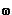) ) and complex admittance
( Y() ) are given by the equations below. (Ref 1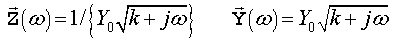Yo has the same definition as for the Warburg impedance. Yo can be used to calculate the diffusion coefficient for the mobile species using the same equations as for the Warburg. The high frequency region can be used to estimate Yo.More About EIS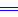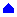More About ... The Warburg Circuit ElementCalculating Diffusion CoefficientsFitting EIS DataMore About EIS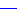REFERENCES(1) "Equivalent Circuit (EQUIVCRT.PAS) Users Manual", Boukamp, B, Univ. Twente, Enschede, the Netherlands, 1989.(2) "Impedance Studies on Chromite-Titanate Porous Electrodes under Reducing Conditions", Gonzalez-Cuenca, Zipprich, Boukamp, Pudmich, Tietz, Fuel Cells, 1 (2001), 256-264.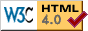© Copyright 2003-2014 Research Solutions & Resources LLC.  All rights reserved.